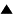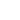Variety# Variety

 Variety is a measure of the number of distinct states a system can be in
The set of all possible states that a system can be in defines its state space. An essential component of cybernetic modelling is a quantitative measure for the size of that state space, or the number of distinct states. This measure is called variety. Variety represents the freedom the system has in choosing a particular state, and thus the uncertainty we have about which state the system occupies. Variety V is defined as the number of elements in the state space S, or, more commonly, as the logarithm to the basis two of that number:

V = log2 (|S|)

The unit of variety in the logarithmic form is the bit. A variety of one bit, V=1, means that the system has two possible states, that is, one difference or distinction. In the simplest case of n binary variables, V = log2(2n) = n is therefore equal to the minimal number of independent dimensions.

## Background

Variety has always been a fundamental idea in Cybernetics and Systems Science, and is so in Metasystem Transition Theory. Variety is defined as a multiplicity of distinctions. The existence of variety is necessary for all change, choice, and information. A reduction in the quantity of variety is the process of selection. If variety has thus been reduced, i.e. if actual variety is less than potential variety, then we say that there is constraint.

Frequently the quantity of variety and the change in the quantity of variety (positive increase or negative decrease) is critical to understand system evolution. Where variety is manifest in a process, then we sometimes want to say that there is uncertainty about the outcome of the process; when that uncertainty is relieved but the occurrence of one of the possibilities, then we gain information. The are many possible ways to measure the quantity of variety, uncertainty, or information. As defined above, the simplest is the count of the number of distinct states. More useful can be the logarithm of that number as a quantity of information, which is called the Hartley entropy. When sets and subsets of distinctions are considered, possibilistic nonspecificities result . The most celebrated are the stochastic entropies of classical information theory, which result from applying probabilistic distributions to the various distinctions.

 HomeMetasystem Transition TheorySystems Concepts Up Prev.Next Down